cancel
Showing results for
Did you mean:
cancel
Showing results for
Did you mean:

## Zone Areas and Volumes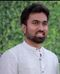Graphisoft

Zone area and volume calculations may seem to be tricky sometimes when you can’t track which areas are calculated exactly and how.In the following I will try to clarify the differences between the area types and why they may or may not show up when you would expect them to do so.

Let’s start with a quick recap. If you have gone through the relevant Help Center articles on Graphisoft websites then you already know that there are:

• 2 automatic methods for placing the Zones:
• Inner Edge
• Reference Line
• the latter having two display options:
• Net
• Gross
• 4 types of area calculations:
• Measured
• Measured Net
• Reduced
• Calculated
• which should depend on the Zone preferences under Options/Project Preferences/Zones… and
• 4 options for elements to set their Relations to Zones:
• No effect on Zones
• Zone Boundary
• Reduce Zone Area only
• Subtract from Zones

Yet the whole thing doesn’t seem to work out if you quickly place a column into a small room for example. Regardless of its size, the column area will not be listed under the reductions, so what’s the point of having the Zone preferences then? Not to mention that Net Area equals Measured Area as well…Let’s take a look at the zone and element options/settings above in details.

## Placement methods

This is fairly simple, the Inner Edge will detect the net areas (Measured Net Area), in this case we cannot expect the gross area to be different from Measured Area.

The Reference Line method however will detect the gross areas up to the reference lines. (Yes, this may not include the whole gross area). It will also detect the net area.

The Net/Gross display options are only available for the Reference Line method (even though the placement of the radioboxes may indicate that these can be used for all methods). This is merely to pick how the Zone will be displayed on the plan when it is selected.## Areas

By definition the Measured Area should be treated as gross area, the Measured Net Area as net, the Reduced Area should include the reductions from the net area as per the Zone preferences and the Calculated Area should include additional reductions that are defined by the percentage value at the Reduced by field.

So how to fix the Reduced area part?

## ‘Reduce Zone Area only’

I have created a simple example in which we can track the area calculations. I used only the default settings for the elements (Relation to Zones is Zone Boundary) and in the Zone preferences, unless otherwise stated.

In order to distinguish the net and gross areas the Reference Line method was used for the Zone placement.

The only exception is the free-standing Wall, which is set with Reduce Zone Area only: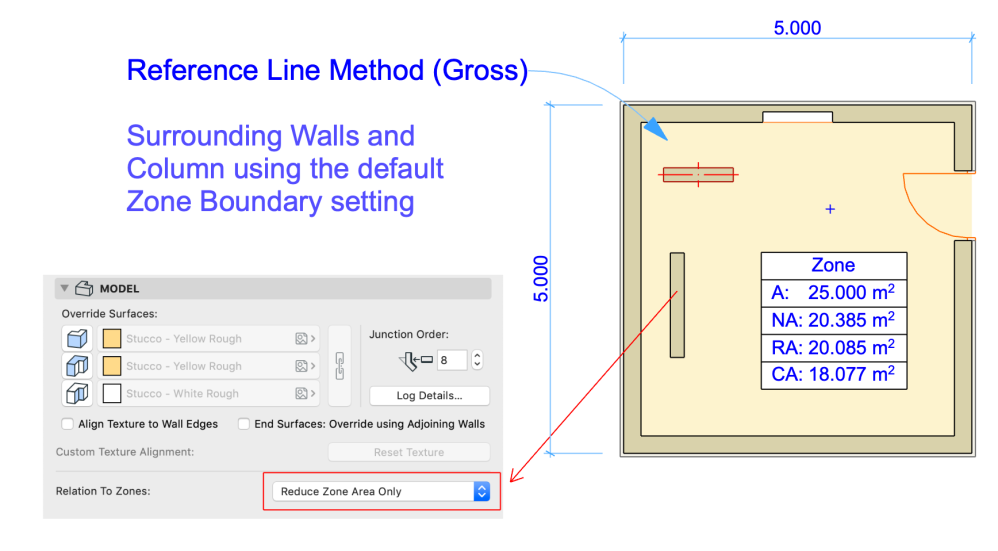And thats’ it! You can see that now the Measured, the Measured Net and the Reduced Areas are differentiated and this is represented in the Zone Settings dialog as well (0.3 m2 is subtracted from the Net Area – this is the Wall area only).

The column (as a Zone Boundary still) is subtracted as part of the Net Area calculation as is not reflected in the area subtraction:What’s more, this reduction is now based on the Zone preferences:

The Wall is 1500 mm long and 200 mm thick, therefore its area is 0.3 m2. Changing the wall thickness to 50 mm, its area would be 0.075 m2 only, which is below the defined minimum of 0.1 m2 in the Zone preferences, therefore it would not be subtracted. (Update Zones after changing the geometry, otherwise the changes will not be reflected in the numbers!)

If the relation is the default ‘Zone Boundary’ then the Net Area will have it deducted already, therefore it will not show up as Reduced Area. On one hand it is making things simpler, you just have to ignore the Reduced Area – and give up on having an area breakdown.

The Reduce by field is used as an example only, it has no relation to the previous subtractions.

How about the Wall niche at the wall on the top? The niche is a full height recess, starting from the floor, therefore should affect the area calculation.

The surrounding wall is set as a Zone Boundary still, but the niche will be automatically excluded when calculating the Net Area, along with the openings.

If the niche area is reduced to be below the size that is set in the Zone preferences then it will be ignored.

Note that openings and niches are not even listed in the area subtractions in the Zone settings.

So why not having everything as Reduce Zone Area only instead of the Zone Boundary? You will see later that because it affects the volume calculation as well and I will go into that too shortly. Till then here is the breakdown of the area calculations of the example above: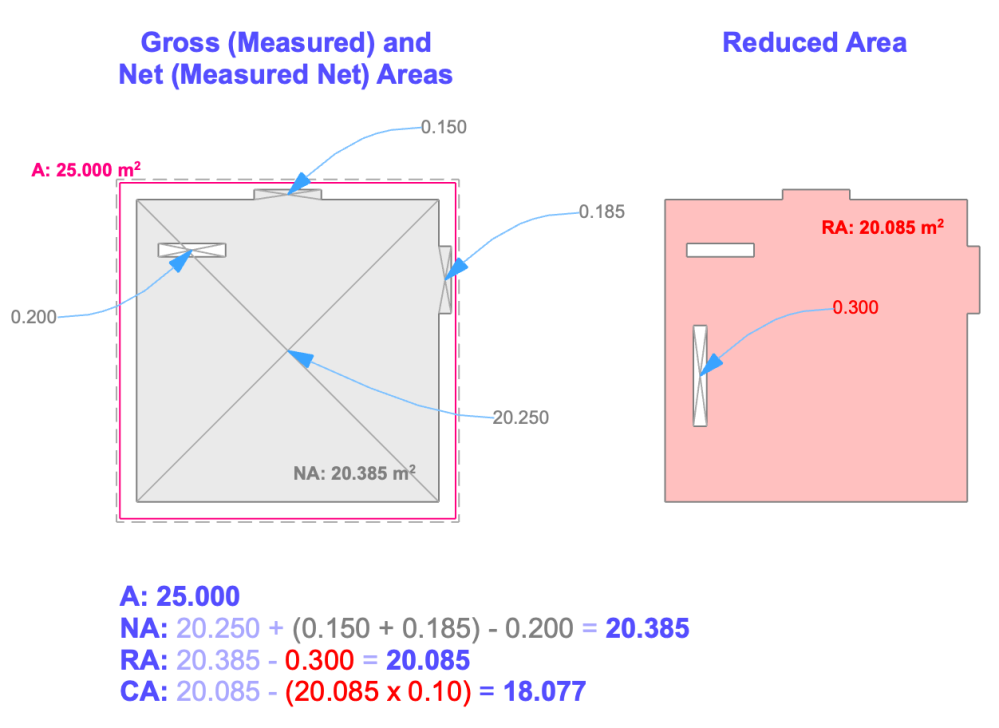## Pros and Cons of ‘Relation to Zones’ settings

Going back to the question why shouldn’t we just use Reduce Zone Area only setting for all elements, find here a comparison of the different options on another example. I indicated pros with green, cons with red, kind of neutral effects with orange:The Zone is 5 m x 5 m, making it 25 m2. This is only the area within the walls. Net Area may be different if the column area is subtracted. Net Area and Reduced Area may be the same or differ, depending on the Relation settings. Volumes are also depending on this. Note that the Zone is 3 m high, the Column is only 2 m.

Doesn’t make it easier to choose which option is the best, right? The right choice really depends on the situation, I would leave the Zone Boundary whenever possible and use Subtract from Zones or Reduce Zone Area only if I need to show the area breakdown and after deciding how accurate the volume calculation needs to be.

Zone Boundary may generate simplified volumes (for example if the column in this example is not full height, then the volume above will be subtracted as well – but this difference may also be ignored if agreed) and does not provide area breakdown for Reduced Area.

In case of exporting the Zones for MEP calculations, Subtract from Zones will give the precise volumes, but there is no area breakdown.

If the breakdown is more important, but the volume is not, then use Reduce Zone Area only.

See this whole thing from the volume’s side:Note the difference between Zone Boundary and Subtract from Zones. The latter will subtract the exact volumes, while Zone Boundary removes everything at full height where areas are excluded from the zone areas.

Reduce Zone Area only and No effect on Zones simply work as their names suggest, both will ignore the volumes of the column.

To summarize this with numbers (Zone is 25 m2 and 75 m3, Column is 0.25 m2 and 0.50 m3):## Low-ceiling Areas

Almost done, now we just need to take a look at low-ceiling areas – how will roofs, suspended ceilings and related connections, such as Trim and SEO affect the calculations.Let’s start with the Roof first. The angle was set graphically on the section so that it is easy to track which areas would be subtracted and the Zone is connected using Trim to Roof. The two height values that are given in the Zone preferences are 1 and 2 metres under which we want to subtract 100% and 50% of the areas respectively.

The distance of these boundaries is measured from the side.The Zone is set with Reference Line method to show the difference between Measured and Net Areas. A regular Fill was also added – I would like to subtract the area that it covers from the Zone, therefore the Subtract from Zones checkbox is checked and a percentage is specified (100%).

The red dashed lines are projected from the section and indicate the measured distances from the side – just to know which areas will be subtracted. Note that the Fill area and the low-ceiling area overlaps a bit too, so it will be interesting to see how this is going to be handled. Will it be subtracted twice or is there an order?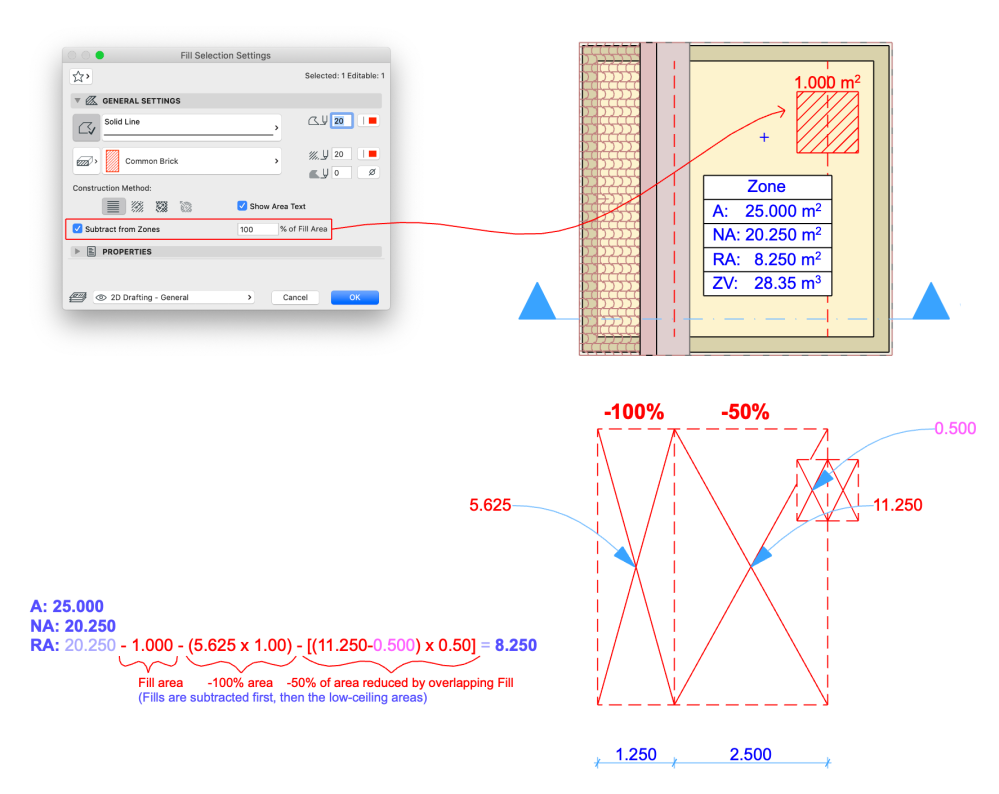There is! Just as it is in the Area Calculation panel, first the fill will be removed, then the remaining areas under the Roof:Trimming will automatically affect the volume calculation.

Let’s see the suspended ceiling example as well. The ceiling is modeled with the Slab and the side is with the Wall tool. Based on the Zone preferences 50% of this area should be subtracted as the remaining height under the ceiling is between 1 and 2 metres.

The Zone is connected using SEO Subtraction with upward extrusion, where the Wall and the Slab are the operators. (Wall could be excluded since the Slab covers that part as well.)On the plans comes an important change. The sidewall of the ceiling box needs to be modified. If you leave it as Zone Boundary, the whole area under the ceiling will be ignored as the plan view will handle the wall as a full height wall when defining the Zone Boundary. So which option to choose? I’m not interested in the Wall to affect the area breakdown, because the Wall is not on the floor and the low-ceiling reduction should handle the area below the Slab, but it would be nice to exclude the volume of the Wall, so I chose Subtract from Zones.Volumes will exclude the SEO subtracted parts automatically.

## Conclusion

Hope the above helps to clear the mystery around the Zone calculations. As you can see the subtractions are happening even if you just use Zone Boundary relations and look for the Net Areas, but if you need an area breakdown (displayed and scheduled) or need more precise volumes you may want to change to another relation setting.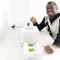This is a very powerful thread, Vimal!